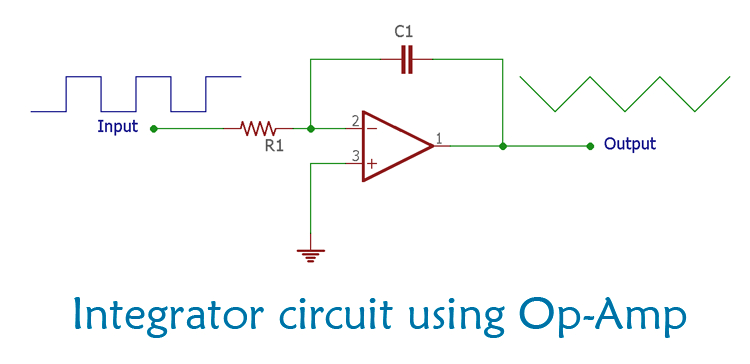# Operational Amplifier Integrator Circuit: Cons…## Operational Amplifier Integrator Circuit: Construction, Working and Applications

In simple Op-Amp applications, the output is proportional to the input amplitude. But when op-amp is configured as an integrator, the duration of the input signal is also considered. Therefore, an op-amp based integrator can perform mathematical integration with respect to time. The integrator produces an output voltage across the op-amp, which is directly proportional to the integral of the input voltage; therefore the output is dependent on the input voltage over a period of time.

Here is the full tutorial: https://circuitdigest.com/tutorial/op-amp-integrator-circuit-working-construction-applications